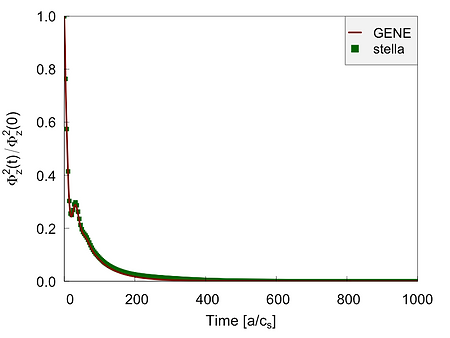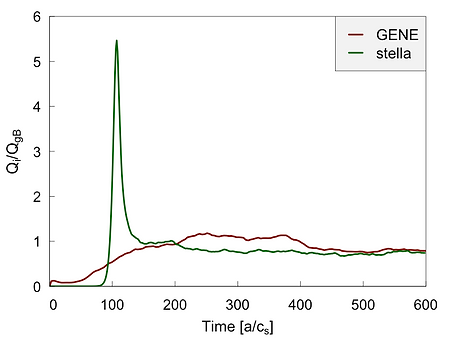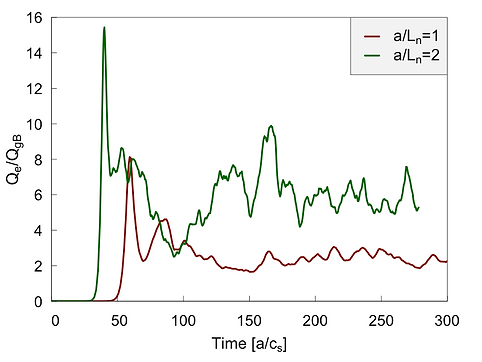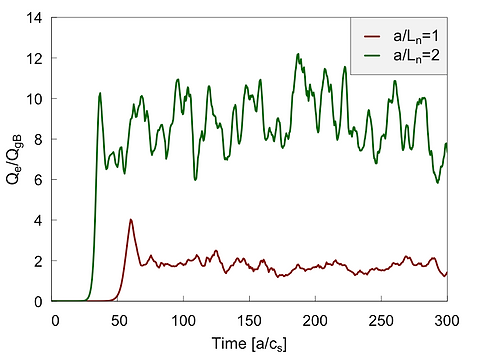top of page

## Flux surface simulations with stella and GENE codes

Flux-surface simulations

## Task: We compare ITG growth rates between stella and GENE for a W7-X (high-mirror) vacuum configuration. Input: s=0.25, a/LTi=3, a/Ln=0, Te=Ti, adiabatic electrons, ρ*=0.00441968 Note that ρ* for GENE is defined as ρ/a (a= VMEC minor radius) and ρ=cs/Ωi  Here, cs does not contain        .## Equilibrium:## W7-X## We compare growth rates between stella and GENE for a linear ITG instability. In the stella calculation, we notice excitation of spurious modes at small scales (kyρ > 8.5), as shown in the figure below (negative growth rates excluded):

Spurious modes## We focus on the wavenumbers kyρ=4.7 (left) associated to the maximum growth rate and ky ρ=9.7 (right) corresponding to the maximum spurious growth rate, and plot the evolution of the growth rate with respect to the stella iterations:## It is clearly seen that the spurious mode fails to converge and fluctuates around 0. The strongest mode, on the other hand, quickly converges to the resulting growth rate. To remedy the spurious mode, we increase nstep by doubling it from 3e4 to 6e4. However, as the following figure suggests, this method does not resolve the issue:Memory testing

Benchmark linear

# Linear Simulations: We simulate ITG with adiabatic electrons. The normalized ion temperature gradient is                    and the density gradient is                    . The comparison for the growth rates and frequencies is shown below:# Next, we show ITG with kinetic electrons, the gradients remain as before, and in addition we set                      and The comparison for the growth rates and frequencies is shown below:# Zonal flow response: We use                   . The comparison for the squared zonal potential is shown below:# Nonlinear Simulations: We simulate ITG with adiabatic electrons. The normalized ion temperature gradient is                  and the density gradient is                    . The comparison for the ion heat fluxes rates is shown below:Benchmark NL

# Next, we show ITG with kinetic electrons, the gradients remain as before, and in addition we set                      and The comparison for ion and electron heat fluxes is shown below:# In addition, we show the comparison for the particle flux:# Testing the parallel b.c. We present the comparison for ITG turbulence simulations for stella, using either the twist and shift boundary condition or the periodic boundary condition.# Relating ITG heat flux with max-J. In the following stella simulations we apply                    plus a density gradient. We use the vacuum W7-X configurations: ref_128 (mr=8%) and ref_404 (mr=-4%), also a tokamak as reference case. The surface for the simulations is r/a=0.75.# Formally, only W7-X mr=8% is max-J. However, we may characterize the configurations according to a degree of max-J. For instance, mr=8% has a higher degree than mr=-4%, which in turn has a higher degree than TOK. We should then expect that the ITG ion heat fluxes respond to the density gradient according to that degree: for TOK, the ion heat flux is enhanced by the density gradient, for mr=-4% the heat flux is almost unchanged, and for mr=8% the ion heat flux is reduced.# mr=+8%# mr=-4%# TOKbottom of page